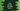# Write a number guessing game in Java## Number guessing game in Java:

In this post, we will write one number guessing game. The program will take one number as input from the user for a number of times and try to match with a secret number. For our program, it will try for 5 times. If any guess in these 5 attemps matches with the secret number, the user will win.

### Algorithm to use:

We will use the below algorithm:

1. Create one random number and keep it in a variable.
2. Run one infinite loop.
3. Take one number as input from the user.
4. Check the current attemp number. If it is 5, end the game, and also print the number. Else, compare the number with the secret number.
5. If the input value is not equal, print one message describing it is less or more.
6. If it is equal to the number, print one message that the number is correct, and end the game.

### Number guessing Java program:

Below is the complete program:

``````import java.util.Scanner;

public class Main {
public static void main(String args[]) {
int totalCount = 1;
int userInputNumber = 0;

Scanner inputScanner = new Scanner(System.in);
int resultNumber = (int) (Math.random() * 100 + 1);

while (true) {
System.out.println(totalCount+"/5");
System.out.print("Enter a number between 1 to 100: \n");
userInputNumber = inputScanner.nextInt();

if (userInputNumber < 1 || userInputNumber > 100) {
System.out.println("Please enter a valid number");
continue;
} else if (totalCount == 5) {
System.out.println("Maximum attempt reached! Please try again...Correct number is : " + resultNumber);
break;
} else if (userInputNumber < resultNumber) {
System.out.println("It is smaller than the result !");
} else if (userInputNumber > resultNumber) {
System.out.println("It is greater than the result !");
} else {
System.out.println("It is correct. You Won !");
break;
}

totalCount++;
}
}
}``````

Here,

• totalCount is the total number of attempts user did. It is initialised as 1.
• userInputNumber is a variable to store the user input value.
• resultNumber is a random number created before the program starts. It can be any number in 1 to 100.
• The while loop runs for infinite number of times.
• Inside the loop, it asks for the user to enter a number. It reads the number and keeps it in userInputNumber.
• If it is an invalid value, it asks the user to enter it again. If the value of totalCount is 5, i.e. user has reached the maximum attempt. It ends the game, i.e. breaks from the while loop.
• If the input value is greater or less than the final number, it prints one message.
• Else, i.e. if the user input number is equal to the secret number, it print one message that this is the correct value and exits from the loop.

### Sample output:

If you run the above program, it will print output as like below:

``````1/5
Enter a number between 1 to 100:
333
Please enter a valid number
1/5
Enter a number between 1 to 100:
100
It is greater than the result !
2/5
Enter a number between 1 to 100:
50
It is greater than the result !
3/5
Enter a number between 1 to 100:
30
It is greater than the result !
4/5
Enter a number between 1 to 100:
20
It is greater than the result !
5/5
Enter a number between 1 to 100:
10
Maximum attempt reached! Please try again...Correct number is : 7

Process finished with exit code 0``````